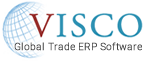(845) 383-3800SEE THE SOFTWAREREQUEST A DEMOCONTAINER TRACKING

# How to Calculate Landed Costs

## How to Calculate Landed Costs

Companies that import and distribute goods have more to consider in their Cost of Goods Sold calculation than just the cost of the material itself.  Typical Landed cost calculations will include

• Material Cost
• Duty
• Ocean Freight
• Drayage
• Customs Broker Fees
• Port Exam Fees
• Commissions

Some companies will also include warehouse costs in the landed cost calculation although calculating this is a challenge so estimating Warehouse charges or just considering them overhead expenses usually makes more sense.

In calculating Gross Profit per sale companies will include Outbound freight costs although most do not consider this an element of the landed cost.

To calculate your landed cost per unit you would take the sum of all “Inventoriable costs” (see above) and divide by your total quantity to see how much each unit really costs.

There will be some costs like Ocean Freight which apply to an entire container.  If that container has multiple items in it, then deciding how much of the cost each item gets requires some additional calculation.  If you buy and sell in weights (LB for example) then you will want to take the total weight of all items on the container to figure out the freight cost per LB, then you multiply the weight of each item x the freight cost per LB to calculate how much of the ocean freight each item should get.

For Example:

Ocean Freight=\$4000

Item A=25000 LBS

Item B=15000 LBS

25000 + 25000=40000 total LBS on the Container/\$4000 total Ocean Freight Cost= \$.10 per LB

Item A receives \$2500 worth of Ocean Freight Cost

Item B receives \$1500 worth of Ocean Freight Cost

If you buy and sell in Units (PCS for example) then you will likely want to distribute freight or other container related costs based on volume.   This calculation requires you to know either the CBM per unit or the CBM per case/PCS per Case.  To Calculate the Ocean Freight Each Item should get you take the total Ocean Freight Cost/Total CBM for that shipment to come up with the Cost per CBM, then you multiply the Cost per CBM by each items total CBM to come up with the freight cost each item should get.  For example

Ocean Freight Cost=\$4000

Item A=1 CBM per case and 24 PCS per Case or .0417 CBM per Unit

Item B=.5 CBM per case and 36 PCS per Case or .0139 CBM per unit

Container has 30 Cases of Item A and 40 Cases of Item B

Total CBM for the container=(30 x 1 CBM per case) + (40 x .5 CBM per Case)=50 CBM

Total Freight Cost per CBM=\$4000/50= \$80 per CBM

Freight Cost for Item A= \$80 X 1=\$80 per Case or \$80/24=\$3.33 per unit

Freight Cost for Item B \$80 X .5=\$40.00 per Case or \$40.00/36=\$1.11 per unit

It is important to accurately calculate your landed costs so you can set appropriate sale price on an item to allow for a reasonable mark up while staying competitive.

BLOG CATEGORIES
Related Articles